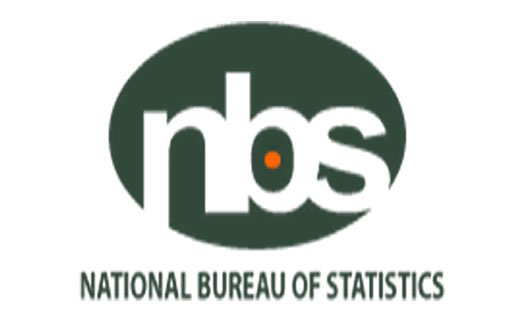N2.5 trillion worth of goods imported into Nigeria in 3 monthsThe National Bureau of Statistics, NBS, says total imports of goods into the country in the first quarters of 2018 is valued at N2.518 trillion, which is 19.2 percent higher than the fourth quarters of 2017, valued at N2.112 trillion and 8.04% higher than the first quarters of 2017, valued at N2.33 trillion.

A report by NBS said the value of imported agricultural goods in the first quarter of 2018 was N184.49 billion, which declined by 18.90% from the fourth quarters of 2017, put at N227.49 billion and by 5.87% from first quarters of 2017, put at N196 billion.

Also, the report revealed that raw materials imports in the first quarters of 2018 was N284.81 billion, representing a growth by 1.93% compared to the fourth quarters of 2017 (N279.41 billion) and by 9.89% compared to first quarters of 2017 (N259.17 billion).

Solid minerals imports in the first quarters of 2018 is put at N12.62 billion, which  decreased by 17.27% on a quarter-to-quarter basis (N15.26 billion), however increased by 3.67% on a year-to-year basis (N12.17 billion).

Energy goods imports in the first quarters of 2018 is N32.4 million, which is 76.51% lower than the fourth quarters of 2017 (N138.1 million), and 205.28% higher than the first quarters of 2017 (N10.6 million).

SHARE